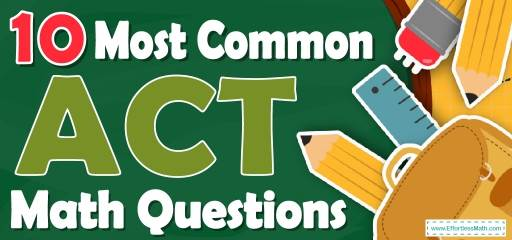# 10 Most Common ACT Math QuestionsPreparing for the ACT Math test? Want a preview of the most common mathematics questions on the ACT Math test? If so, then you are in the right place.

The mathematics section of ACT can be a challenging area for many test-takers, but with enough patience, it can be easy and even enjoyable!

Preparing for the ACT Math test can be a nerve-wracking experience. Learning more about what you’re going to see when you take the ACT can help to reduce those pre-test jitters. Here’s your chance to review the 10 most common ACT Math questions to help you know what to expect and what to practice most. Try these 10 most common ACT Math questions to hone your mathematical skills and to see if your math skills are up to date on what’s being asked on the exam or if you still need more practice.

Make sure to follow some of the related links at the bottom of this post to get a better idea of what kind of mathematics questions you need to practice.

## 10 Sample ACT Math Practice Questions

1- A company pays its employer $7000 plus $$2\%$$ of all sales profit. If $$x$$ is the number of all sales profit, which of the following represents the employer’s revenue? A. $$0.02x$$ B. $$0.98x-7000$$ C. $$0.02x+7000$$ D. $$0.98x+7000$$ E. $$-0.98x-7000$$ 2- What is the area of a square whose diagonal is 8 cm? A. 16 $$\space cm^2$$ B. 32 $$\space cm^2$$ C. 34 $$\space cm^2$$ D. 128 $$\space cm^2$$ E. 256 $$\space cm^2$$ 3- What is the value of $$x$$ in the following figure? A. 150 B. 145 C. 125 D. 105 E. 95 4- Right triangle ABC is shown below. Which of the following is true for all possible values of angles A and B? A. tan A = tan B B. sin A = cos B C. tan$$^2$$ A=tan$$^2$$ B D. tan A=1 E. cot⁡ A=sin B 5- What is the value of y in the following system of equation? $$3x-4y= -20$$ $$-x+2y= 10$$ A. $$-1$$ B. $$-2$$ C. 1 D. 4 E. 5 6- How long does a 420–miles trip take moving at 50 miles per hour (mph)? A. 4 hours B. 6 hours and 24 minutes C. 8 hours and 24 minutes D. 8 hours and 30 minutes E. 10 hours and 30 minutes 7- From the figure, which of the following must be true? (figure not drawn to scale) A. $$y = z$$ B. $$y = 5X$$ C. $$y≥x$$ D. $$y+4x=z$$ E. $$y>x$$ 8- Which is the correct statement? A. $$\frac{3}{4} > 0.8$$ B. $$10\% = \frac{2}{5}$$ C. $$3 <\frac{5}{2}$$ D. $$\frac{5}{6} > 0.8$$ E. None of the above 9- When $$40\%$$ of 60 is added to $$12\%$$ of 600, the resulting number is: A. 24 B. 72 C. 96 D. 140 E. 160 10- A ladder leans against a wall forming a 60$$^\circ$$ angle between the ground and the ladder. If the bottom of the ladder is 30 feet away from the wall, how long is the ladder? A. 30 feet B. 40 feet C. 50 feet D. 60 feet E. 120 feet ## Best ACT Math Prep Resource for 2022 ## Answers: 1- C Employer’s revenue: $$0.2x+7000$$ 2- B The diagonal of the square is 8. Let $$x$$ be the side. Use Pythagorean Theorem: $$a^2 + b^2 = c^2$$ $$x^2 + x^2 = 82 ⇒ 2x^2 = 82 ⇒ 2x^2 = 64 ⇒x^2 = 32 ⇒x= √32$$ The area of the square is: $$√32 × √32 = 32$$ 3- B $$x=20+125=145$$ 4- B By definition, the sine of an acute angle is equal to the cosine of its complement. Since angle A and B are complementary angles, therefore: sin A = cos B 5- E Solve the system of equations by elimination method. $$3x-4y= -20$$ $$-x+2y=10$$ Multiply the second equation by 3, then add it to the first equation. $$3x-4y= -20$$ $$3(-x+2y=10)$$ $$\Downarrow$$ $$3x-4y= -20$$ $$-3x+6y=30)$$ ⇒ add the equations $$2y=10⇒y=5$$ 6- C Use distance formula: $$Distance = Rate × time ⇒ 420 = 50 × T$$, divide both sides by 50. $$420 / 50 = T ⇒ T = 8.4$$ hours. Change hours to minutes for the decimal part. 0.4 hours $$= 0.4 × 60 = 24$$ minutes. 7- D $$x$$ and $$z$$ are colinear. $$y$$ and $$5x$$ are colinear. Therefore, $$x+z=y+5x$$,subtract $$x$$ from both sides,then,$$z=y+4x$$ 8- D Check each option. A. $$\frac{3}{4} > 0.8$$ $$\frac{3}{4}$$ =0.75 and it is less than 0.8. Not true! B. $$10\% = \frac{2}{5}$$ $$10\% = 1/10<\frac{2}{5}$$. Not True! C. $$3 < \frac{5}{2}$$ $$\frac{5}{2} =2.5<3$$. Not True! D. $$\frac{5}{6} > 0.8$$ $$\frac{5}{6}$$ =0.8333… and it is greater than 0.8. Bingo! E. None of them above Not True! 9- C $$40\%$$ of 60 equals to: $$0.40×60=24$$ $$12\%$$ of 600 equals to: $$0.12×600=72$$ $$40\%$$ of 60 is added to $$12\%$$ of $$600: 24+72=96$$ 10- D The relationship among all sides of special right triangle 30$$^\circ$$-60$$^\circ$$- 90$$^\circ$$ is provided in this triangle: In this triangle, the opposite side of 30$$^\circ$$ angle is half of the hypotenuse. Draw the shape of this question: The latter is the hypotenuse. Therefore, the latter is 60 ft. ## College Entrance Tests Looking for the best resource to help you succeed on the ACT Math test? ## The Best Books to Ace the ACT Math Test ## Related to This Article ### More math articles ### What people say about "10 Most Common ACT Math Questions - Effortless Math: We Help Students Learn to LOVE Mathematics"? No one replied yet. X 52% OFF Limited time only! Save Over 52% SAVE$40

It was $76.99 now it is$36.99